Succeed with maths – Part 1

Start this free course now. Just create an account and sign in. Enrol and complete the course for a free statement of participation or digital badge if available.

Free course

1.3 Writing a fraction as a percentage

Using the same idea that multiplication by 100 per cent means multiplication by 1, you can also convert fractions into percentages.

So if you want to convert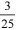to a percentage, it can be shown as follows: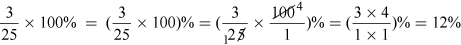As an alternative, you can turn the fraction into a decimal (by dividing the numerator by the denominator) and then move the decimal point two places to the right (because you are multiplying by 100). This is shown in the example below, where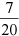is converted to a percentage.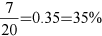As with a lot of maths, there are other alternative ways to convert a fraction into a percentage. You can also multiply the fraction by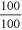, as shown in this example: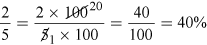For this last example, note that the fraction can be expanded by multiplication so that the denominator is turned into 100. For some fractions, this gives you another alternative for the conversion. For this last example, it looks like this: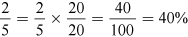You’ve covered a few ideas here, so here’s a quick summary as a reminder before you have a go yourself:

• Percentage to fraction – write the percentage value as a fraction out of 100, and simplify.
• Percentage to a decimal – divide the percentage value by 100.
• Decimal to percentage – multiply the decimal by 100 per cent.
• Fraction to percentage – multiply the fraction by 100 per cent, and simplify as necessary.

You may be wondering why any of this matters! It is not just some interesting maths; being able to carry out these conversions can help you when it comes to working with percentages in problems.

Bear all these ‘rules’ in mind as you work through this week.